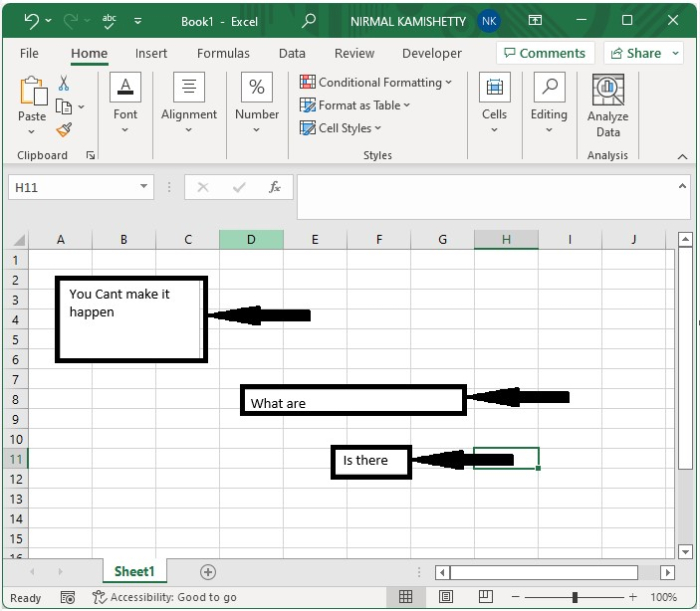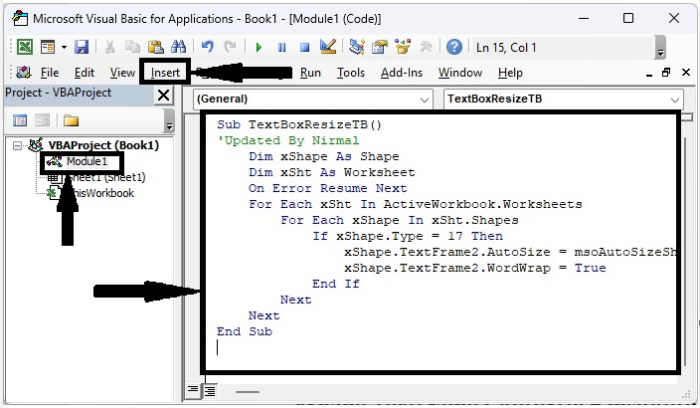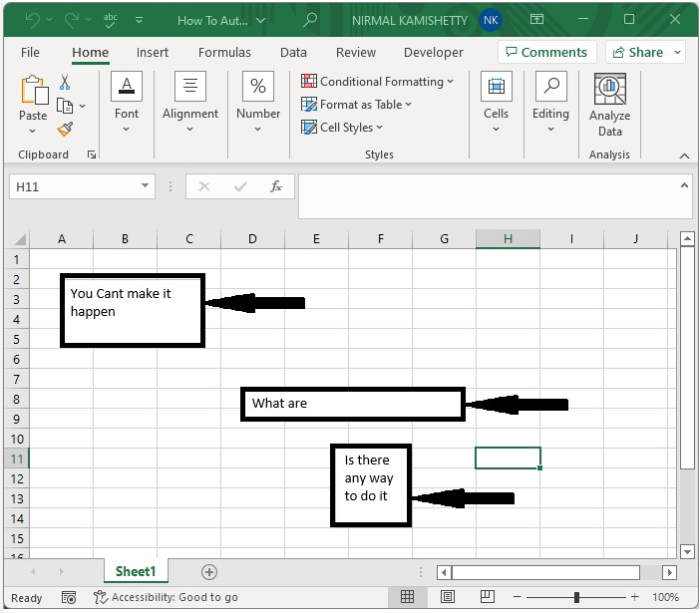# How to Automatically Resize the Textbox to Fit the Contents in Excel?

When we use the text boxes in Excel, you may have observed that manually resizing the text boxes to fit the content is one of the most time-consuming processes, as adjusting them could be a slower process. This can waste a significant amount of time when doing the Excel work. This tutorial will help you understand how we can automatically resize the text box to fit the content in Excel. We can do it with the help of a VBA application, as it cannot be done by default in Excel.

## Automatically Resize a Textbox to Fit the Content in Excel

Here, we will first insert a VBA module and then run it to complete our task. Let us see an effortless process to know how we can automatically resize the text box to fit content in Excel.

Step 1

Let us consider an excel sheet where it contains text boxes, as shown in the below excel sheet as an image. We can insert a text box by clicking on text under "Insert" and drawing the box's size.Now right-click on the sheet name and select view code to open the vba application, then click on Insert and select the module, then enter the programme into the text box as shown in the below image.

### Example

Sub TextBoxResizeTB()
'Updated By Nirmal
Dim xShape As Shape
Dim xSht As Worksheet
On Error Resume Next
For Each xSht In ActiveWorkbook.Worksheets
For Each xShape In xSht.Shapes
If xShape.Type = 17 Then
xShape.TextFrame2.AutoSize = msoAutoSizeShapeToFitText
xShape.TextFrame2.WordWrap = True
End If
Next
Next
End Sub


The code will only work for increasing the text size it won’t reduce the text size.Step 2

Now save the sheet as a macro-enabled workbook, and then click on F5 to run the module and auto-resize the text boxes as shown in the below image.## Conclusion

In this tutorial, we used a simple example to demonstrate how we can automatically resize a textbox to fit its contents in Excel.

Updated on: 11-Jan-2023

186 Views